# Graph Classification Classification Outline Introduction Overview Classification using

• Slides: 33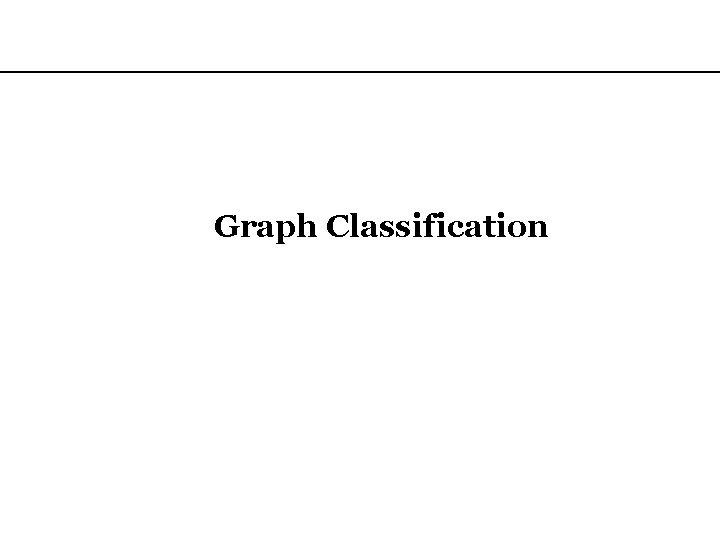Graph ClassificationClassification Outline • Introduction, Overview • Classification using Graphs – Graph classification – Direct Product Kernel • Predictive Toxicology example dataset – Vertex classification – Laplacian Kernel • WEBKB example dataset • Related Works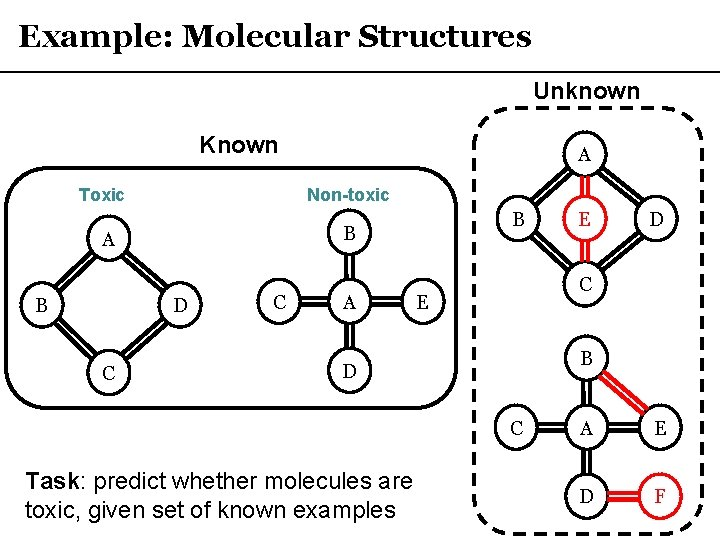Example: Molecular Structures Unknown Known Toxic Non-toxic D C B B A C A D C E B D C Task: predict whether molecules are toxic, given set of known examples E A E D F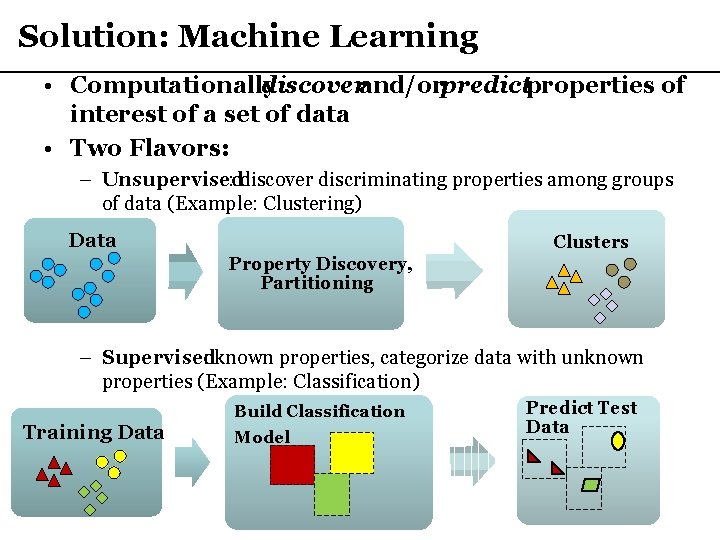Solution: Machine Learning • Computationally discoverand/or predictproperties of interest of a set of data • Two Flavors: – Unsupervised : discover discriminating properties among groups of data (Example: Clustering) Data – Clusters Property Discovery, Partitioning – Supervised : known properties, categorize data with unknown properties (Example: Classification) Training Data Build Classification Model Predict Test DataClassification · Classification : The task of assigning class labels in a discrete class la set Y to input instances in an input space X · Ex: Y = { toxic, non-toxic }, X = {valid molecular structures } Misclassified data instance (test error) Unclassified data instances Training the classification model using the training data Assignment of the unknown (test) data to appropriate class labels using the model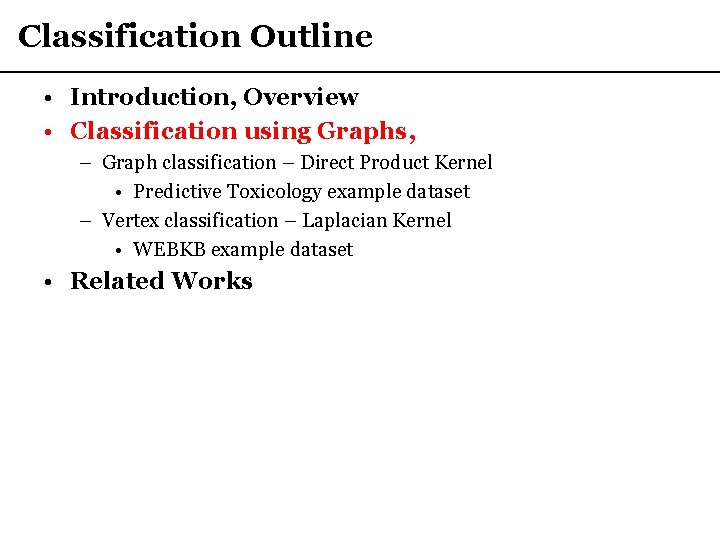Classification Outline • Introduction, Overview • Classification using Graphs, – Graph classification – Direct Product Kernel • Predictive Toxicology example dataset – Vertex classification – Laplacian Kernel • WEBKB example dataset • Related WorksClassification with Graph Structures • Graph classification (between-graph) – Each full graph is assigned a class label • Example: Molecular graphs A B E D • Vertex classification (within-graph) – Within a single graph, each vertex is assigned a class label • Example: Webpage (vertex) / hyperlink (edge) graphs NCSU domain Faculty C Toxic Course StudentRelating Graph Structures to Classes? • Frequent Subgraph Mining (Chapter 7) – Associate frequently occurring subgraphs with classes • Anomaly Detection (Chapter 11) – Associate anomalous graph features with classes • *Kernel-based methods (Chapter 4) – Devise kernel function capturing graph similarity, use vectorbased classification via the kernel trick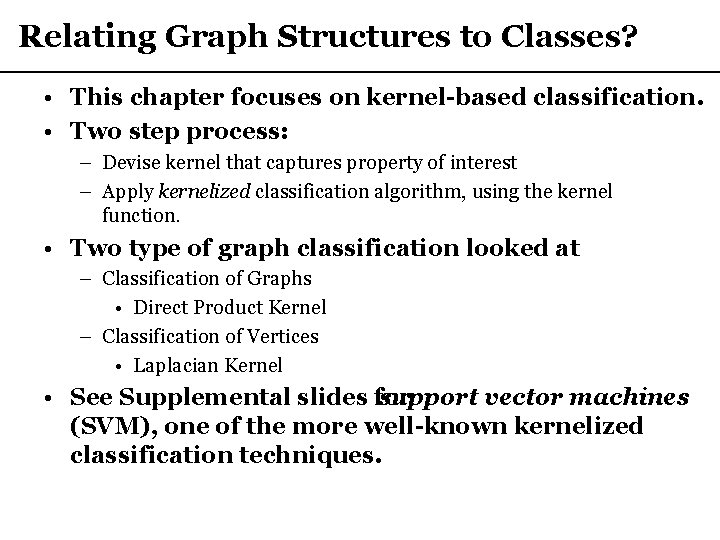Relating Graph Structures to Classes? • This chapter focuses on kernel-based classification. • Two step process: – Devise kernel that captures property of interest – Apply kernelized classification algorithm, using the kernel function. • Two type of graph classification looked at – Classification of Graphs • Direct Product Kernel – Classification of Vertices • Laplacian Kernel • See Supplemental slides for support vector machines (SVM), one of the more well-known kernelized classification techniques.Walk-based similarity (Kernels Chapter) • Intuition – two graphs are similar if they exhibit similar patterns when performing random walks Random walk vertices heavily distributed towards A, B, D, E A B C D E F H I J Random walk vertices heavily distributed towards H, I, K with slight bias towards L Similar! K L Q R S Random walk vertices evenly distributed Not Similar! T U V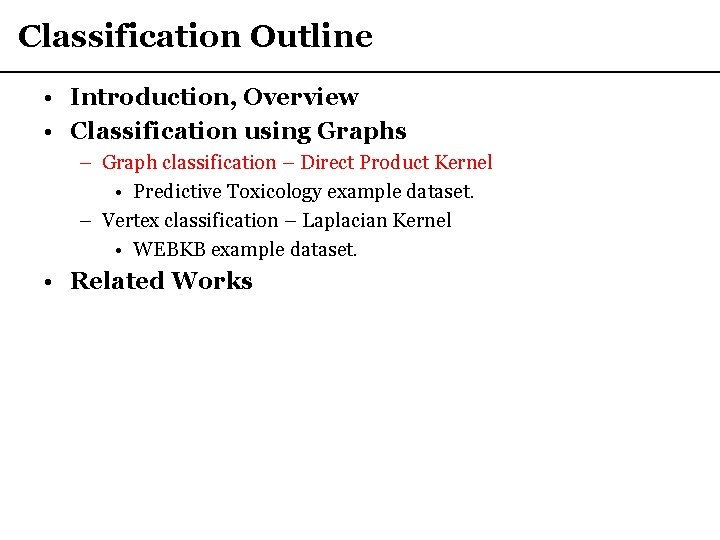Classification Outline • Introduction, Overview • Classification using Graphs – Graph classification – Direct Product Kernel • Predictive Toxicology example dataset. – Vertex classification – Laplacian Kernel • WEBKB example dataset. • Related WorksDirect Product Graph – Formal Definition Input Graphs Direct Product Vertices Direct Product Notation Direct Product Edges IntuitionDirect Product Graph - example B A B D C A C D Type-A Type-B E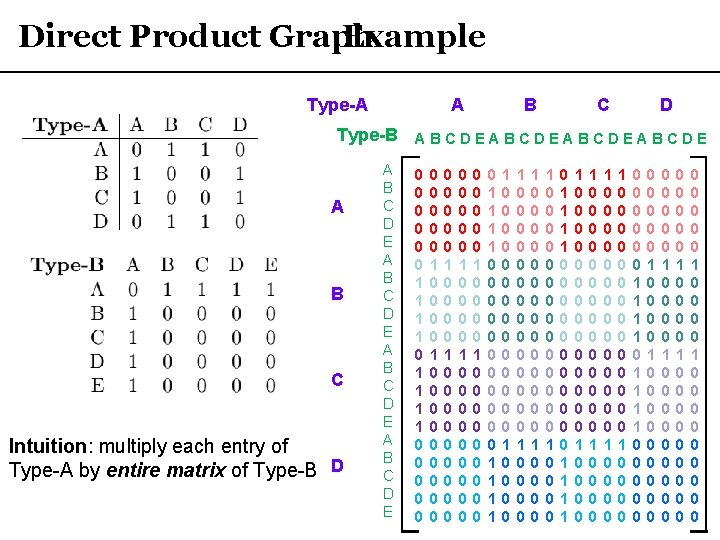Direct Product Graph Example Type-A A B C D Type-B A B C D E A B C Intuition: multiply each entry of Type-A by entire matrix of Type-B D A B C D E 0 0 0 1 1 1 1 0 0 0 0 0 0 0 0 0 0 1 0 0 0 0 1 0 0 0 0 0 0 0 1 1 1 1 0 0 0 0 0 0 1 1 1 1 1 0 0 0 0 0 0 0 0 0 0 0 0 0 0 1 0 0 0 0 1 0 0 0 0 0 0 0 0 0 0 0 0 0 0 1 0 0 0 0 0 1 1 1 1 0 0 0 0 0 0 0 0 0 0 1 0 0 0 0 1 0 0 0 0 0 0 0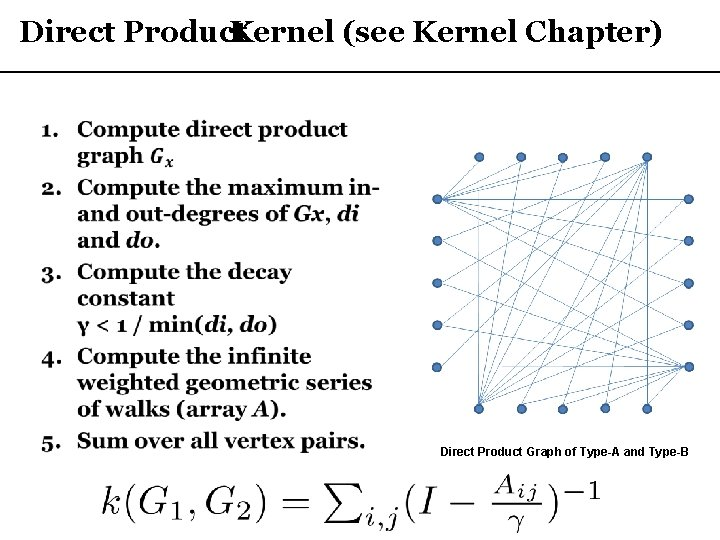Direct Product Kernel (see Kernel Chapter) • Direct Product Graph of Type-A and Type-BKernel Matrix • Compute direct product kernel for all pairs of graphs the set of known examples. • This matrix is used as input to SVM function to create the classification model. • *** Or any other kernelized data mining method!!!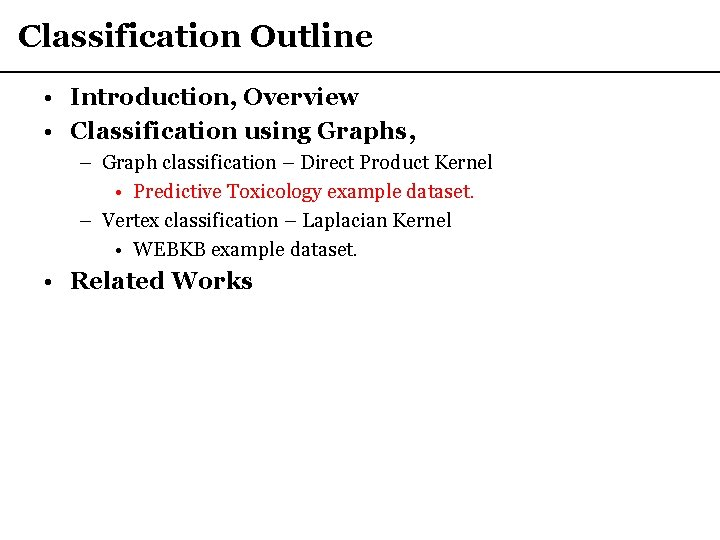Classification Outline • Introduction, Overview • Classification using Graphs, – Graph classification – Direct Product Kernel • Predictive Toxicology example dataset. – Vertex classification – Laplacian Kernel • WEBKB example dataset. • Related WorksPredictive Toxicology (PTC) dataset · The PTC dataset is a collection of molecules that have been tested positive or negative for toxicity. 1. # R code to create the SVM model 2. data(“PTCData”) # graph data 3. data(“PTCLabels”) # toxicity information 4. # select 5 molecules to build model on 5. s. Train = sample(1: length(PTCData), 5) 6. PTCData. Small <- PTCData[s. Train] 7. PTCLabels. Small <- PTCLabels[s. Train] 8. # generate kernel matrix 9. K = generate. Kernel. Matrix (PTCData. Small, PTCData. Small) 10. # create SVM model 11. model =ksvm(K, PTCLabels. Small, kernel=‘matrix’) A B D C B C A D E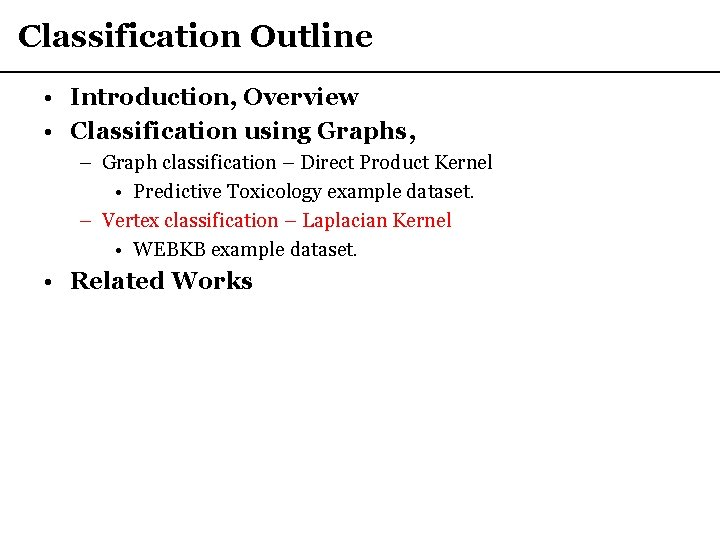Classification Outline • Introduction, Overview • Classification using Graphs, – Graph classification – Direct Product Kernel • Predictive Toxicology example dataset. – Vertex classification – Laplacian Kernel • WEBKB example dataset. • Related Works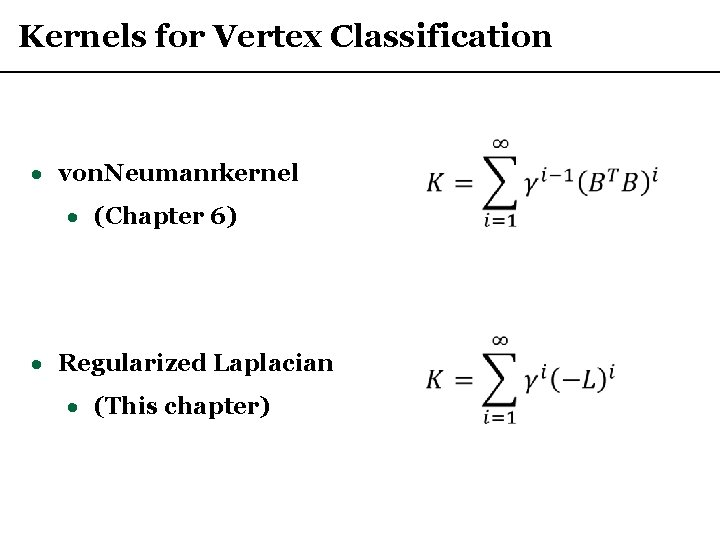Kernels for Vertex Classification · von Neumann kernel · (Chapter 6) · Regularized Laplacian · (This chapter)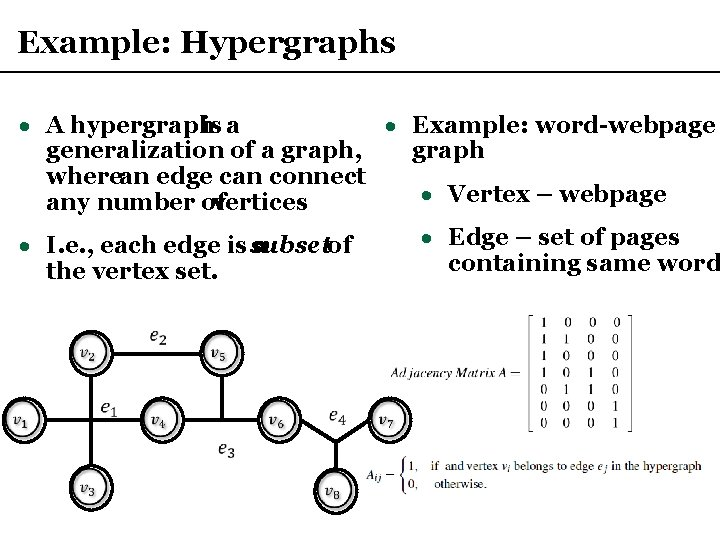Example: Hypergraphs · A hypergraph is a · Example: word-webpage generalization of a graph, graph where an edge can connect · Vertex – webpage any number of vertices · Edge – set of pages · I. e. , each edge is a subset of containing same word the vertex set.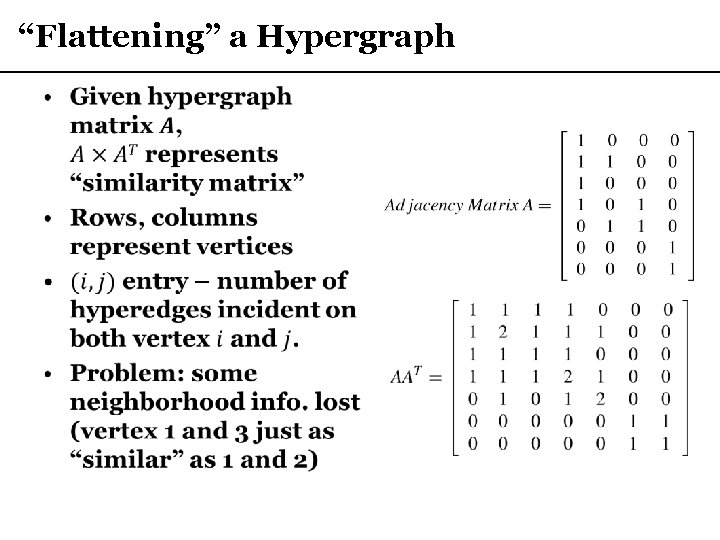“Flattening” a Hypergraph •Laplacian Matrix · In the mathematical field of graph theory the Laplacian matrix (L), is a matrix representation of a graph. · L = D – M · M – adjacency matrix of graph (e. g. , A*AT from hypergraph flattening) · D – degree matrix (diagonal matrix where each (i, i) entry is vertex i‘s [weighted] degree) · Laplacian used in many contexts (e. g. , spectral graph theory)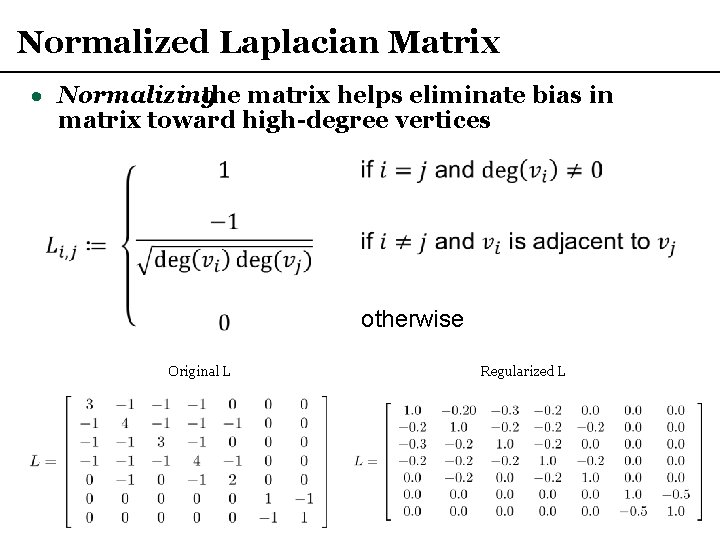Normalized Laplacian Matrix · Normalizing the matrix helps eliminate bias in matrix toward high-degree vertices otherwise Original L Regularized L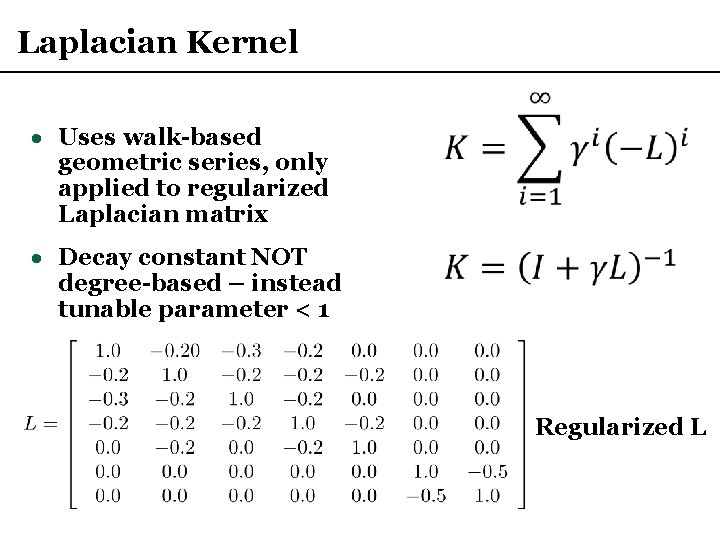Laplacian Kernel · Uses walk-based geometric series, only applied to regularized Laplacian matrix · Decay constant NOT degree-based – instead tunable parameter < 1 Regularized L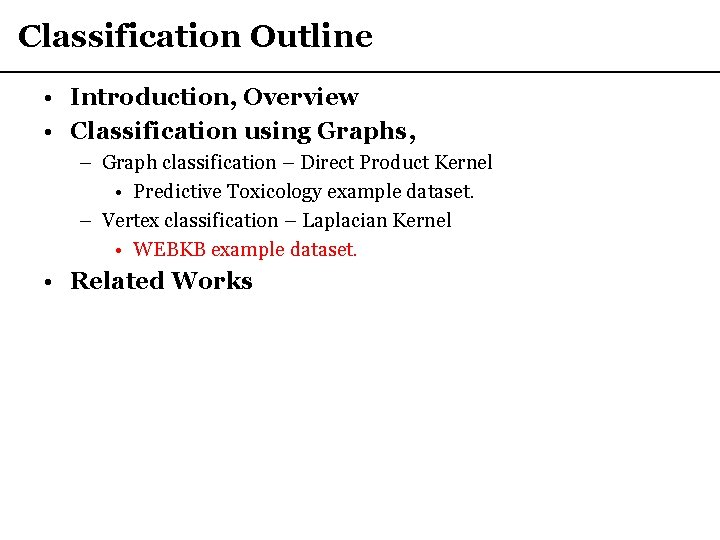Classification Outline • Introduction, Overview • Classification using Graphs, – Graph classification – Direct Product Kernel • Predictive Toxicology example dataset. – Vertex classification – Laplacian Kernel • WEBKB example dataset. • Related Works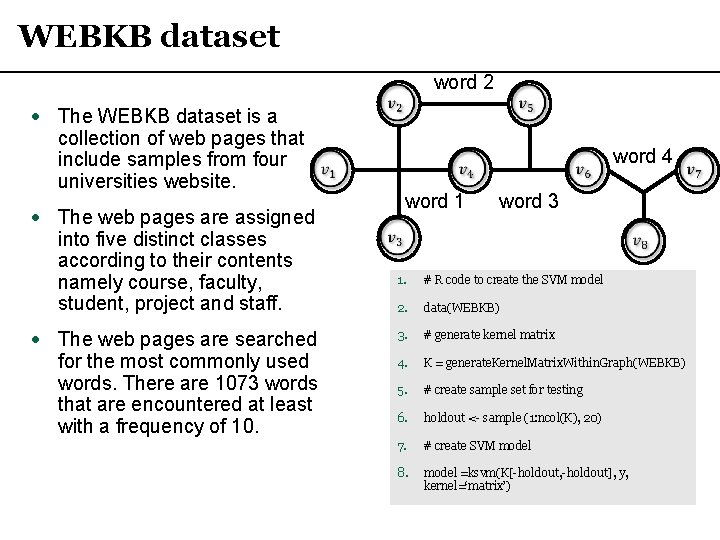WEBKB dataset · The WEBKB dataset is a collection of web pages that include samples from four universities website. · The web pages are assigned into five distinct classes according to their contents namely course, faculty, student, project and staff. · The web pages are searched for the most commonly used words. There are 1073 words that are encountered at least with a frequency of 10. word 2 word 1 word 4 word 3 1. # R code to create the SVM model 2. data(WEBKB) 3. # generate kernel matrix 4. K = generate. Kernel. Matrix. Within. Graph(WEBKB) 5. # create sample set for testing 6. holdout <- sample (1: ncol(K), 20) 7. # create SVM model 8. model =ksvm(K[-holdout, -holdout], y, kernel=‘matrix’)Classification Outline • Introduction, Overview • Classification using Graphs, – Graph classification – Direct Product Kernel • Predictive Toxicology example dataset. – Vertex classification – Laplacian Kernel • WEBKB example dataset. • Kernel-based vector classification – Support Vector Machines • Related WorksRelated Work – Classification on Graphs • Graph mining chapters: – Frequent Subgraph Mining (Ch. 7) – Anomaly Detection (Ch. 11) – Kernel chapter (Ch. 4) – discusses in detail alternatives to the direct product and other “walk-based” kernels. • g. Boost – extension of “boosting” for graphs – Progressively collects “informative” frequent patterns to use as features for classification / regression. – Also considered a frequent subgraph mining technique (similar to g. Span in Frequent Subgraph Chapter). • Tree kernels – similarity of graphs that are trees.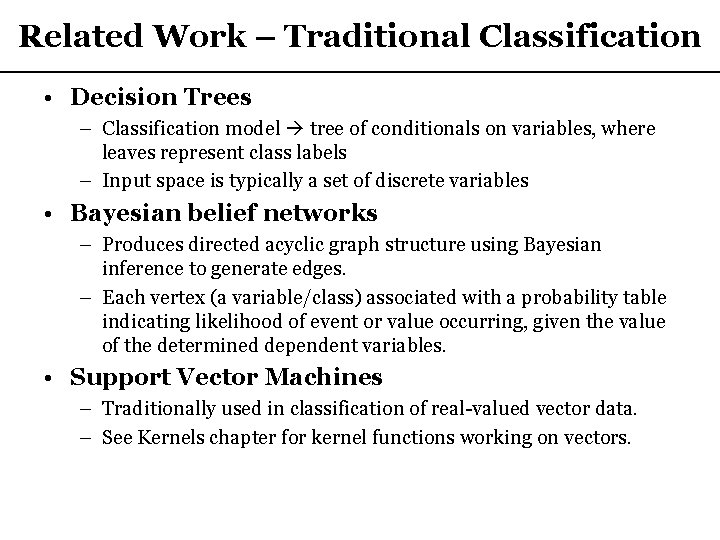Related Work – Traditional Classification • Decision Trees – Classification model tree of conditionals on variables, where leaves represent class labels – Input space is typically a set of discrete variables • Bayesian belief networks – Produces directed acyclic graph structure using Bayesian inference to generate edges. – Each vertex (a variable/class) associated with a probability table indicating likelihood of event or value occurring, given the value of the determined dependent variables. • Support Vector Machines – Traditionally used in classification of real-valued vector data. – See Kernels chapter for kernel functions working on vectors.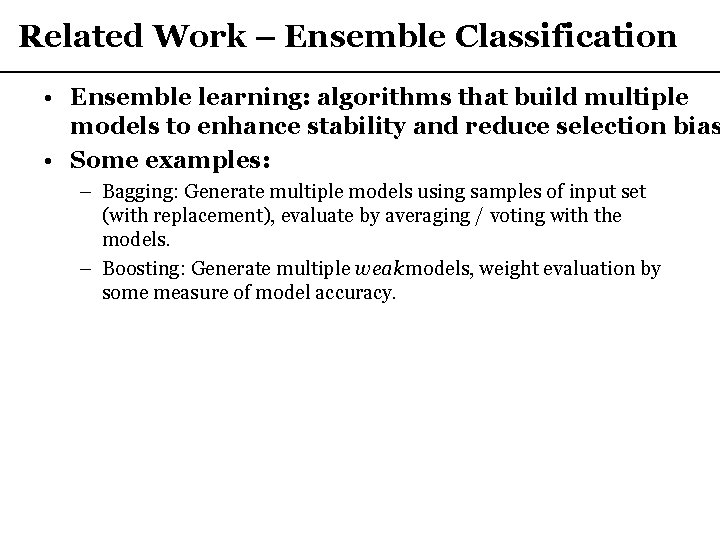Related Work – Ensemble Classification • Ensemble learning: algorithms that build multiple models to enhance stability and reduce selection bias • Some examples: – Bagging: Generate multiple models using samples of input set (with replacement), evaluate by averaging / voting with the models. – Boosting: Generate multiple weak models, weight evaluation by some measure of model accuracy.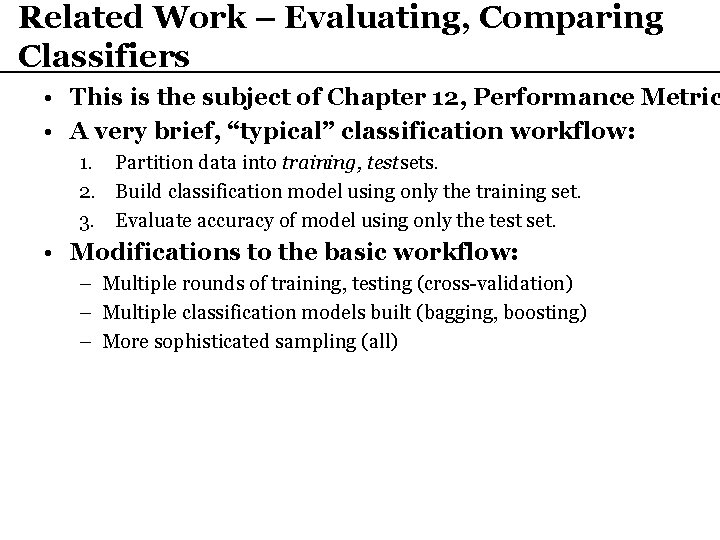Related Work – Evaluating, Comparing Classifiers • This is the subject of Chapter 12, Performance Metric • A very brief, “typical” classification workflow: 1. Partition data into training, test sets. 2. Build classification model using only the training set. 3. Evaluate accuracy of model using only the test set. • Modifications to the basic workflow: – Multiple rounds of training, testing (cross-validation) – Multiple classification models built (bagging, boosting) – More sophisticated sampling (all)Related Work – Evaluating, Comparing Classifiers • This is the subject of Chapter 12, Performance Metric • A very brief, “typical” classification workflow: 1. Partition data into training, test sets. 2. Build classification model using only the training set. 3. Evaluate accuracy of model using only the test set. • Modifications to the basic workflow: – Multiple rounds of training, testing (cross-validation) – Multiple classification models built (bagging, boosting) – More sophisticated sampling (all)